Tools:
Distance calculator – Find the distance between two maidenhead squares
Unit converter – Convert between units
Dipole antenna – Calculate the optimal lengths of a dipole antenna for a given frequency
Vertical antenna – Calculate the optimal lengths of a vertical antenna for a given frequency
Cubical quad antenna – Calculate the optimal lengths of a cubical quad antenna for a given frequency
Inverted vee antenna – Calculate the optimal lengths of an inverted vee antenna for a given frequency
Ground plane antenna – Calculate the optimal lengths of a ground plane antenna for a given frequency
J pole antenna – Calculate the optimal lengths of a J pole antenna for a given frequency
VSWR – Convert between VSWR, Refection coefficient, return loss and mismatch loss
Three element Yagi antenna – Calculate the optimal lengths of a three element Yagi antenna for a given frequency
Seven element Yagi antenna – Calculate the optimal lengths of a seven element Yagi antenna for a given frequency
Wavelength frequency converter – Convert between wavelength and frequency
Air core inductor – Calculate the inductance or lengths of an air core inductor
Low pass filter – Calculate the units of an RC or RL LPF circuit
High pass filter – Calculate the units of an RC or RL HPF circuit
Ohm’s law – Convert between voltage, current, resistance and power by entering two
Reactance – Calculate the reactance of a capacitor or inductor
Decibel calculator – Convert between decibel values or calculate dB by entering the power or voltage
Voltage divider – Calculate the output voltage or resistance of one resistor
Resistor Color codes – Find the resistance of a resistor by entering the colors of the bands
Resistors in parallel – Calculate the resistance of resistors in parallel
Resistors in series – Calculate the resistance of resistors in series
Capacitors in parallel – Calculate the capacitance of capacitors in parallel
Capacitors in series – Calculate the capacitance of capacitors in series
Coaxial cable – Calculate the properties of a coaxial cable
Inductors in series – Calculate the inductance of inductors in series
Inductors in parallel – Calculate the inductance of inductors in parallel
Full wave loop antenna – Calculate the optimal lengths of a full wave loop antenna for a given frequency
ERP and EIRP – Calculate and convert ERP and EIRP
Half wave coax balun – Calculate the optimal length of a half wave coax balun for a given frequency
Small magnetic loop – Calculate the optimal lengths of a small magnetic loop for a given frequency
Narrowband balun – Calculate the inductance and capacitance of a narrowband balun
Pi attenuator – Calculate the values of the resistors by entering the attenuation and impedance
T attenuator – Calculate the values of the resistors by entering the attenuation and impedance

What’s New
VSWR calculator with forward power and reflected power
Removed millihertz to avoid confusion
Bug fixes

Screenshots

•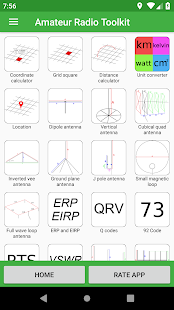••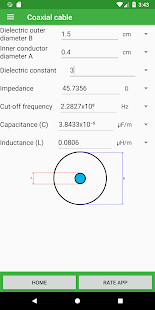•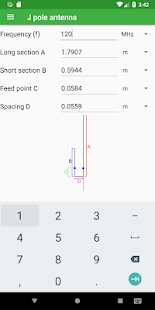•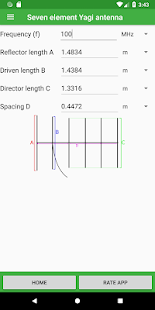•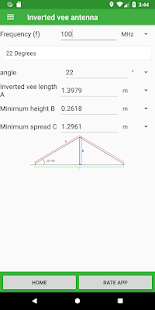••